Time: 3 Hours                                                                                                     Max. Marks: 100

NOTE: There are 9 Questions in all.

·      Question 1 is compulsory and carries 20 marks. Answer to Q. 1. must be written in the space provided for it in the answer book supplied and nowhere else.

·      Out of the remaining EIGHT Questions answer any FIVE Questions. Each question carries 16 marks.

·      Any required data not explicitly given, may be suitably assumed and stated.

Q.1       Choose the correct or best alternative in the following:                                         (2x10)

a.       In a metal, the conductivity is very large because

(A)  the mobility of charge carriers is very high.

(B)  the number of charge carriers is very high.

(C)    positive as well as negative charge carriers contribute to current.

(D)   all electrons in the metal contribute to the conduction current.

b.      In a laser, the following processes have to be minimized

(A)    Absorption only.

(B)    Absorption and spontaneous emission.

(C)    Absorption and stimulated emission.

(D)    Stimulated emission only.

c.   In a tunnel diode oscillator, the diode bias is

(A)    small and forward.                        (B)  large and forward.

(C)  Zero.                                            (D)  Reverse.

d.   The band gap Eg in a semiconductor corresponds to the energy difference between

(A)    The top of the conduction band and the top of the valence band.

(B)    The top of the conduction band and the bottom of the valence band.

(C)  The bottom of the conduction band and the top of the valence band.

(D)  The bottom of the conduction band and the bottom of the valence band.

e.   Make the choice of the correct one from the following statements:

(A)     Direct recombination is normally radiative.

(B)     All radiation is due to direct recombination.

(C)     Both of the above statements are true.

(D)    Neither of the above statements are true.

f.    Crystal defects in a semiconductor tends to

(A)     Reduce majority carrier concentration in a semiconductor.

(B)     Reduce equilibrium minority carrier concentration in a semiconductor.

(C)     Increase the rate of indirect recombination in a semiconductor.

(D)    Increase radiative recombination in a semiconductor.

g.   The low field electron mobility depends on

(A)     Doping.                                        (B)  Electric field.

(C)  Temperature.                                (D)  None of the above.

h.   If the quasi-fermi levels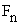and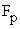are such thatlies above, it indicates that

(A)    excess carriers are present in the semiconductor.

(B)    the semiconductor is n type.

(C)    the semiconductor is p type.

(D)    the region under consideration is in depletion.

i.    Under reverse bias, the capacitance of the junction

(A)   Reduces with increasing applied voltage.

(B)   Increases with increasing applied voltage.

(C) is independent of applied bias.

(D) First increases then decreases with increasing magnitude of applied bias.

j.    The potential well formation in a CCD structure occurs when the surface of the semiconductor is in

(A)  Deep depletion.                            (B)  Strong inversion.

(C)  Weak inversion.                           (D) Accumulation.

Answer any FIVE Questions out of EIGHT Questions.

Each question carries 16 marks.

Q.2     a.   Sketch the conduction and valence bands before and after diffusion of carriers in a PN junction.                                                                 (8)

b.   For what voltage will the reverse current in p-n junction Ge diode reach 90% of its saturation value at the room temperature?  What is the ratio of the current for a forward bias of 0.05V to the current for same magnitude reverse bias?                                                                                     (8)

Q.3     a.   Discuss the variation in semiconductor parameters with temperature.                   (8)

b.   Explain the continuity equation and its significance.                                              (8)

Q.4     a.   Discuss metal-semiconductor junctions.  How are they different from semiconductor-semiconductor junctions?  Explain with examples.        (8)

b.   Determine the conversion efficiency of a solar cell of input power              = 900 mW, open circuit voltage = 600 mV, short circuit current density      =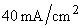, fill factor = 75% and area = 6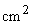.       (8)

Q.5     a.   Describe Early effect and punch through in practical BJT devices.                       (8)

b.   Explain short channel effects in MOSFETs.                                                        (8)

Q.6     a.   Discuss the differences between transferred electron devices and avlanche transit time devices.                                                                  (12)

b.   A Gunn diode has a drift length of 2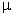m.  What minimum voltage is required to initiate the Gunn mode?                                                                 (4)

Q.7     a.   Discuss the characteristics of microwave transistors.                                         (12)

b.   Calculate the bandwidth of an LED which has a rise time of 20nsec.                    (4)

Q.8     a.   Describe various steps of IC fabrication process.                                                (8)

b.   Compare and contrast monolithic and hybrid circuits.                                          (8)

Q.9     a.   The Hall coefficient of a certain silicon specimen was found to be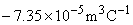from 100 to 400 K.  Determine the nature of the semiconductor.  Further the electrical conductivity was found to be         200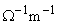.  Calculate the density and mobility of charge carriers.                         (8)

b.   Explain the various capacitances associated with p-n junctions.                           (8)